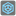• # Test 5 Issue

## Question related to missionCount Inversions

My code is successful on the first 4 tests but gets 2635 for test 5 instead of 4181 on the 5th test. Help is greatly appreciated!

```def count_inversion(sequence):
"""
Count inversions in a sequence of numbers
"""
length = len(sequence)
count, x, y = 0, 0, 1
while y < length:
if sequence[x] > sequence[y]:
z = y + 1
count += 1
while z < length:
if sequence[x] > sequence[z]:
count += 1
z += 1
x += 1
y += 1
print('Final count =', count)
return count
```4